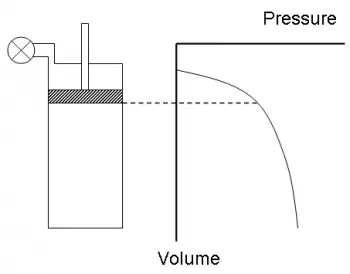# What Is a Thermodynamic State?

A thermodynamic state is a set of variables of a thermodynamic system that must be specified in order to reproduce the system. The individual parameters are known as state variables, state parameters, or thermodynamic variables.When a sufficient set of thermodynamic variables has been specified, the values ​​of all other system properties are defined. The number of values ​​needed to establish the state depends on the system and is not always known.

## What Is a State Function?

State functions describe the momentary condition of a thermodynamic system. State functions depend only on the system's state, regardless of the path used to go from the initial to the final state. It means that incremental changes in these variables are exact differentials.

Some examples of state functions are

• Enthalpy.

• Entropy.

• Internal energy.

• Pressure.

• Temperature.

• Volume.

These values ​​can be both intensive and extensive magnitudes and are independent of the path taken to reach the current state.

System state functions generally have a particular dependency on each other. The dependence between thermodynamic functions is universal.

Several thermodynamic diagrams serve to model the transitions between thermodynamic states.

Each system or type of substance is characterized by an equation of state or constitutive equation that relates some of the state variables to each other. This is because systems in equilibrium have a finite number of degrees of freedom according to the Gibbs phase rule.

### Equation of State of an Ideal Gas

The most straightforward equation of state is the one that describes the behavior of a gas in a closed system when it is at low pressure and high temperature. Under these conditions, the gas density is very low, and we can make the following approximations:

• There are no interactions between the gas molecules.

• The volume of the molecules is zero.

The equation of state that describes a gas under these conditions is called the ideal gas equation of state.

The equation of state for an ideal gas is the result of combining two empirical laws valid for very dilute gases: Boyle's law and Charles' law .

For one mole of gas, the constant that appears on the second side of the above equation is the universal ideal gas constant R, so the ideal gas equation of state is:

P·V = n·R·T

Where,

• P is the pressure

• V is the volume

• n is the number of moles.

• R in the International System is 8.31 J/mol·K

## What Is a Path Function?

A path function is a physical quantity that describes the transition of a system between two equilibrium states.

For example, heat and work are process quantities since they quantitatively describe the transition between equilibrium states of thermodynamic systems.

In a reversible process, it is not only a function of the initial and final states but also of the successive intermediate states through which the system goes through (if the process were not reversible, it would be necessary to specify in detail how it is carried out). In this case, id depends on the path.

## State of Thermodynamic Equilibrium

Systems found in nature are often dynamic and complex.

In many cases, their states can be described as approaching ideal conditions.

One of these ideal conditions is the equilibrium state. The laws of thermodynamics postulate that all isolated systems will tend to change in order to approach the equilibrium state.

The equilibrium state is a primitive object of classical or equilibrium thermodynamics, in which it is called a thermodynamic state.

There are several different types of equilibrium states, corresponding to different physical variables. A system reaches thermodynamic equilibrium when the conditions of all relevant types of equilibrium are satisfied simultaneously.

Listed below are a few different kinds of balance.

• Thermal equilibrium: When a system is in thermal equilibrium, the temperature throughout a system is uniform and the system is in thermal equilibrium.

• Mechanical equilibrium: if at each point within a given system there is no change in pressure and no movement of material.

• Phase Equilibrium: This occurs when the mass for each individual phase reaches a value that does not change with time.

• Chemical Equilibrium: In chemical equilibrium, the chemical composition of a system has stabilized and does not change with time.

## What Is a Thermodynamic Process?

A thermodynamic process is the evolution of specific thermodynamic properties relative to a particular thermodynamic system. From the point of view of thermodynamics, these transformations must occur from an initial equilibrium state to a final one. Hence, the magnitudes that undergo a variation when passing from one state to another must be perfectly defined in said initial and final states.

In this way, thermodynamic processes can be interpreted as the result of the interaction of one system with another after some bond has been removed between them, so that finally, the systems are in equilibrium (mechanical, thermal and/or material) with each other.

Less abstractly, a thermodynamic process can be seen as the changes of a system, from some initial conditions to other final conditions, due to its destabilization.

Author:

Published: July 10, 2019
Last review: January 22, 2022